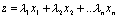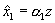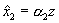# Annex Weighting - More Details for the Technically Minded

This section describes in more detail the principal component weighting process that we use. Let the variables that make up the index be denoted i= 1,..n, and the years for which data is available be denoted t=1,..T, and let the countries for which data is available be denoted j=1,…m. Then let X be the data matrix. It has n columns, one for each of the variables, and in the ith column, there are mT elements, so X has mT rows. The first T elements are the observations on variable i in country 1 in years t=1,2..T, the next T elements are the observations on variable i in country 2 in years t=1,2..T, and so on. Let xi be the ith column of X.

Suppose that the globalisation index for any country and year is constructed by attaching a weight to the ith variable of λi and summing the weighted variables (as we do). Then the value of the index for each country and year is given by a mT x 1 vector z, where(1)

Now – and here is the clever part - the index z can be regarded as an approximation to each of the i=1,..n variables that are used to construct it. In particular, we can approximate x1 by some scalar multiple α1 of z, x2 by some scalar multiple α1 of z, and so on. So, if we denote the approximation to xi as, we have,,…..(2)

We want these approximations to be as good as possible. The goodness of fit measure used in the principal component approach is the sum of squared deviations(3)

where l is an index that runs over all mT observations for a given variable (such as the trade-GDP ratio).

So, the “optimal weighting” problem is to choose weights λ1, .. λn and α1,.. αn to minimise S in (3) subject to (1) holding - which defines z – and (2) holding - which defines the approximations. The solution to the problem therefore chooses weights which make z a best approximation to all of the variables x1,..xn simultaneously, subject to z being a linear combination of the same variables.

The solution to this problem is well-known. It is that both λ1, .. λn and α1,.. αn are equal to the principal eigenvector of the n x n matrix X΄X. This principal eigenvector is easily computed in any statistical package – we used Stata. That gives us the weights λ1, .. λn in the Table above. And I think we will stop there!

(if you know enough matrix algebra to have got this far, but are unfamiliar with eigenvalues and eigenvectors, you should consult any textbook on linear algebra, such as Mathematical Methods for Economists, by Steven Glaister).

View this page as a pdf file